30 day free trialstarting from only \$49.95## Support

F.A.Q. & Expert Forums

# What is Digital Signal Processing?

Digital signal processing (DSP) is the mathematical manipulation of an information signal to modify or improve it in some way. It is characterized by the representation of discrete time, discrete frequency, or other discrete domain signals by a sequence of numbers or symbols and the processing of these signals.

• Digital: operating by the use of discrete signals to represent data in the form of numbers
• Signal: a parameter (electrical quantity or effect) that can be varied in such a way as to convey information
• Processing: a series operations performed according to programmed instructions 

The goal of DSP is usually to measure, filter and/or compress continuous real-world analog signals. The first step is usually to convert the signal from an analog to a digital form, by sampling and then digitizing it using an analog-to-digital converter (ADC), which turns the analog signal into a stream of numbers. However, often, the required output signal is another analog output signal, which requires a digital-to-analog converter (DAC). Even if this process is more complex than analog processing and has a discrete value range, the application of computational power to digital signal processing allows for many advantages over analog processing in many applications, such as error detection and correction in transmission as well as data compression.

### Applications of DSP

• Radar and Sonar: 1) target detection – position and velocity estimation, 2) tracking, 3) weather forecast
• Biomedical: analysis of biomedical signals, diagnosis, patient monitoring, preventive health care, artificial organs. Examples: 1) electrocardiogram (ECG) signal – provides doctor with information about the condition of the patient’s heart 2) electroencephalogram (EEG) signal – provides Information about the activity of the brain
• Speech applications: Examples 1) noise reduction – reducing background noise in the sequence produced by a sensing device (microphone) 2 ) speech recognition – differentiating between various speech sounds 3) synthesis of artificial speech – text to speech systems for blind
• Communications: Examples 1) telephony – transmission of information in digital form via telephone lines, modem technology, mobile phones 2) encoding and decoding of the information sent over a physical channel (to optimise transmission or to detect or correct errors in transmission)
• Image Processing: Examples 1) content based image retrieval – browsing, searching and retrieving images from database 2) compression – reducing the redundancy in the image data to optimise transmission / storage 3) image enhancement
• Music Applications: Examples: 1) recording 2)playback 3) Manipulation (mixing, special effects)
• Multimedia: generation storage and transmission of sound, still images, motion pictures Examples: 1) digital TV 2) video conferencing

## Introduction

The analysis of signals is a fundamental problem for many engineers and scientists. Even if the immediate problem is not electrical, the basic parameters of interest are often changed into electrical signals by means of transducers. Common transducers include accelerometers and load cells in mechanical work, EEG electrodes and blood pressure probes in biology and medicine, and pH and conductivity probes in chemistry. The rewards for transforming physical parameters to electrical signals are great, as many instruments are available for the analysis of electrical signals in the time, frequency and modal domains. The powerful measurement and analysis capabilities of these instruments can lead to rapid understanding of the system under study.

## The Time Domain

The traditional way of observing signals is to view them in the time domain. The time domain is a record of what happened to a parameter of the system versus time. For instance, the following Figure shows a simple spring-mass system where we have attached a pen to the mass and pulled a piece  of paper past the pen at a constant rate. The resulting graph is a record of the displacement of the mass versus time, a time domain view of displacement.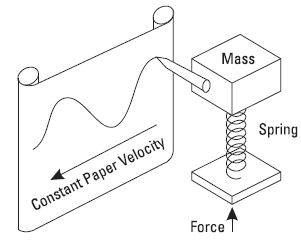Such direct recording schemes are rarely used, but it usually is much more practical to convert the parameter of interest to an electrical signal using a transducer. Transducers are commonly available to change a wide variety of parameters to electrical signals. Microphones, accelerometers, load cells, conductivity and pressure probes are just a few examples.

## The Frequency Domain

It was shown over one hundred years ago by Baron Jean Baptiste Fourier that any waveform that exists in the real world can be generated by ading up sine waves. We have illustrated
this in the following Figure for a simple waveform composed of two sine waves. By picking the amplitudes, frequencies and phases of these sine waves correctly, we can generate a waveform identical to our desired signal.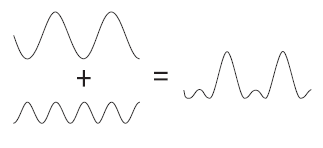This figure can be realized with the following Matlab and XOctave Code

`t = linspace(0,6*pi,100); % timey1 =sin(-t);  % first signaly2=-0.5*cos(2*t);  % second signal% plottingfigure,subplot(2,2,1),plot(t,y1); % top chartsubplot(2,2,3),plot(t,y2), % bottom left chartsubplot(1,2,2),plot(t,y1+y2) % resulting right chart`
` `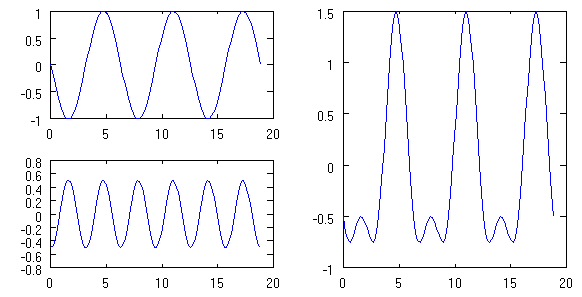Conversely, we can break down our real world signal into these same sine waves. It can be shown that this combination of sine waves is unique; any real world signal can be represented by only one combination of sine waves. The following figure is a three dimensional graph of this addition of sine waves. Two of the axes are time and amplitude, familiar from the time domain. The third axis is frequency which allows us to visually separate the sine waves which add to give us our complex waveform. If we view this three-dimensional graph along the frequency axis we get the view in the following figure. This is the time domain view of the sine waves. Adding them together at each instant of time gives the original waveform.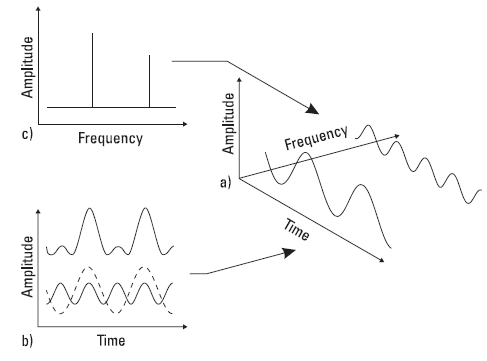The relationship between the time and frequency domains.
a) 3D coordinates showing time, frequency and amplitude b) Time domain view c) Frequency domain view.

Matlab and XOctave code for frequency domain conversion

`dt = t(2)-t(1); % sampling timeFs= 1/dt; %sampling frequencyf1 = fft(y1) / length(y1) * 2 ; % Fast Fourier Transform of signal 1f2 = fft(y2) / length(y2) * 2 ; % Fast Fourier Transform of signal 2f3 = fft(y1+y2) / length(y2) * 2 ; % Fast Fourier Transform of signal 3f1(51:end)=[]; % single side only no need for negative frequenciesf2(51:end)=[];f3(51:end)=[];freq = Fs/2 *linspace(0,1,length(f1)); % single sided frequencyfigure,subplot(2,2,1),bar(freq,abs(f1)); % top chartsubplot(2,2,3),bar(freq,abs(f2)), % bottom left chartsubplot(1,2,2),bar(freq,abs(f3)) % resulting right chart `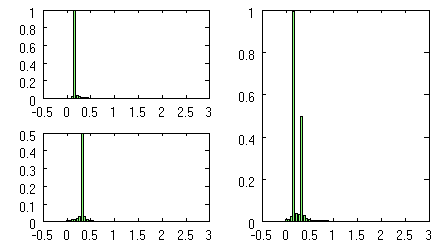At first the frequency domain may seem strange and unfamiliar, yet it is an important part of everyday life. Your ear-brain combination is an excellent frequency domain analyzer. The ear-brain splits the audio spectrum into many narrow bands and determines the power present in each band. It can easily pick small sounds out of loud background noise thanks in part to its frequency domain capability. A doctor listens to your heart and breathing for any unusual sounds. He is listening for frequencies which will tell him something is wrong. An experienced mechanic can do the same thing with a machine. Using a screwdriver as a stethoscope, he can hear when a bearing is failing because of the frequencies it produces.

# References

. Wikipedia : http://en.wikipedia.org/wiki/Digital_signal_processing

. http://www-sigproc.eng.cam.ac.uk

.The Fundamentals of Signal Analysis Application Note 243